Engineering Mechanics

# Engineering Mechanics

Test Description

## 20 Questions MCQ Test GATE Mechanical (ME) 2023 Mock Test Series | Engineering Mechanics

Engineering Mechanics for Mechanical Engineering 2023 is part of GATE Mechanical (ME) 2023 Mock Test Series preparation. The Engineering Mechanics questions and answers have been prepared according to the Mechanical Engineering exam syllabus.The Engineering Mechanics MCQs are made for Mechanical Engineering 2023 Exam. Find important definitions, questions, notes, meanings, examples, exercises, MCQs and online tests for Engineering Mechanics below.
Solutions of Engineering Mechanics questions in English are available as part of our GATE Mechanical (ME) 2023 Mock Test Series for Mechanical Engineering & Engineering Mechanics solutions in Hindi for GATE Mechanical (ME) 2023 Mock Test Series course. Download more important topics, notes, lectures and mock test series for Mechanical Engineering Exam by signing up for free. Attempt Engineering Mechanics | 20 questions in 60 minutes | Mock test for Mechanical Engineering preparation | Free important questions MCQ to study GATE Mechanical (ME) 2023 Mock Test Series for Mechanical Engineering Exam | Download free PDF with solutions
 1 Crore+ students have signed up on EduRev. Have you?
Engineering Mechanics - Question 1

### The co-efficient of friction depends on

Engineering Mechanics - Question 2

### The area moment of inertia about the centroidal axis of a rectangular section is given by 106667 mm4. If the width and depth of the section are 20mm and 40mm, then determine the moment of inertia about the base of the section.

Detailed Solution for Engineering Mechanics - Question 2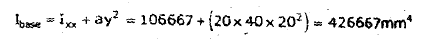Engineering Mechanics - Question 3

### A gear system is used to pull a load of 180 N as shown in figure (All dimensions are in cm). What will be the minimum force required to pull up the load?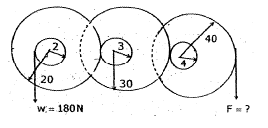Detailed Solution for Engineering Mechanics - Question 3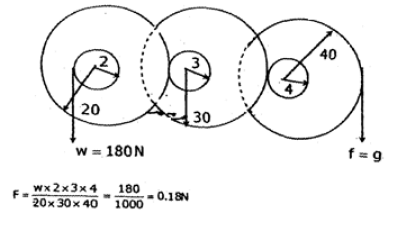Engineering Mechanics - Question 4

A block of mass 5 kg is placed on an inclined plane shows inclination is 30° to ground. Co-efficient of friction between surface and block is 0.3. Find out horizontal force on the block which is required to pull up the block on inclined plane.

Detailed Solution for Engineering Mechanics - Question 4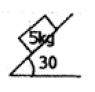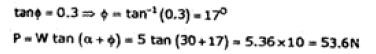Engineering Mechanics - Question 5

In a toggle joint shown in figure, find out horizontal force P. Vertical force at joint C is 5 N. Length of links are 3 m and 5 m.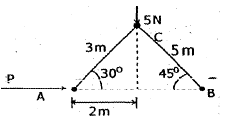Detailed Solution for Engineering Mechanics - Question 5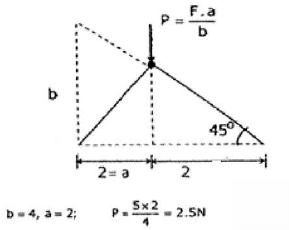Engineering Mechanics - Question 6

Fig. shows the two bodies of masses m1 and m2 connected by a light inextensible string and a passing over a smooth pulley. Mass m2 lies on a smooth horizontal plane.
When mass m1 is moving downward, the acceleration of the two bodies is equal to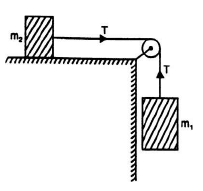Engineering Mechanics - Question 7

The maximum height attained by a ball (of weight 500 N) which is thrown vertically upwards with a velocity 4.9 m/s is equal to

*Answer can only contain numeric values
Engineering Mechanics - Question 8

A tower is of height 100 m. A stone is thrown up from the foot of water with a velocity of 20 m/s and at the same time another stone is dropped from the top of the tower. The two stones will meet after _________ s.

Engineering Mechanics - Question 9

Two weights of 50 N and 150 N (of two blocks A and B respectively) are connected by a string and frictionless and weightless pulleys as shown in Fig. The tension in the string would be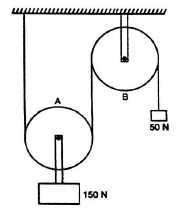*Answer can only contain numeric values
Engineering Mechanics - Question 10

A ladder of weight 250 N is placed against a smooth vertical wall and a rough horizontal floor (µ = 0.3) as shown in Fig.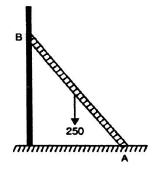If the ladder is on the point of sliding, the reaction at A will be __________ N.

Engineering Mechanics - Question 11

A weight of 200 N is supported by two cables as shown in figure. The tension in the cable making angle θ will be minimum when the value of θ is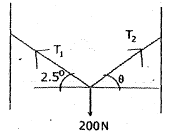Detailed Solution for Engineering Mechanics - Question 11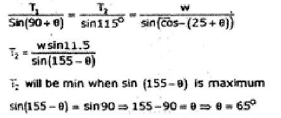Engineering Mechanics - Question 12

T​he three masses of 40 kg, 80 kg and 120 kg are supported as shown in figure. If masses are released simultaneously, then the tight cord will be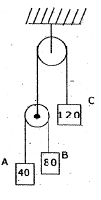Engineering Mechanics - Question 13

A name board of 500 kg is supported by a horizontal bar and inclined cable at 60° as shown in figure. Neglect the weight of bar, tension in cable will be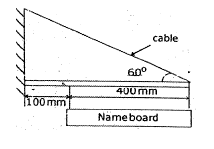*Answer can only contain numeric values
Engineering Mechanics - Question 14

The load ‘W’ for the arrangement shown in figure will be ________ N.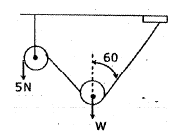Engineering Mechanics - Question 15

In the given figure, find the angle at which the ladder loses its contact with the wall before it hits the ground? Assume centre of mass of ladder moves in a circle and friction less ground and wall.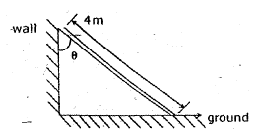Engineering Mechanics - Question 16

Find out force ‘F’ required to pull the weight of 100 N using the pulley as shown in the figure.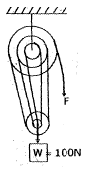Detailed Solution for Engineering Mechanics - Question 16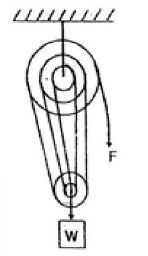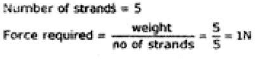Engineering Mechanics - Question 17

Common Data Questions 17-18

In a carnival ride, the passengers sit on a seat that rotates with constant speed in a vertical circle of radius 2 m. The heads of the seated passenger always pointed towards the axis of rotation and the carnival ride completes one full circle in 2 seconds.

Q. Find the acceleration of passenger

Detailed Solution for Engineering Mechanics - Question 17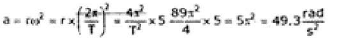Engineering Mechanics - Question 18

In a carnival ride, the passengers sit on a seat that rotates with constant speed in a vertical circle of radius 2 m. The heads of the seated passenger always pointed towards the axis of rotation and the carnival ride completes one full circle in 2 seconds.

Find the slowest rate of rotation if the seat belt is to exert no force on the passenger at the top of ride.

Detailed Solution for Engineering Mechanics - Question 18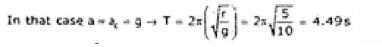Engineering Mechanics - Question 19

A ladder of mass 10 kg leans against a larger smooth sphere of radius 5 m which is fixed on a horizontal surface. The ladder makes an angle 60° with horizontal and having length 12.5 m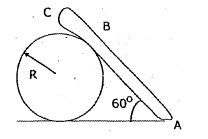What is the force that the sphere exerts on ladder?

Detailed Solution for Engineering Mechanics - Question 19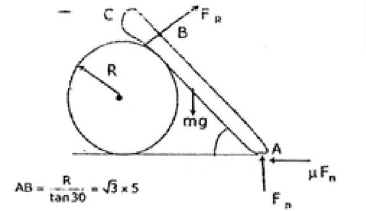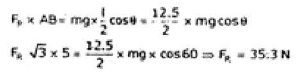Engineering Mechanics - Question 20

A ladder of mass 10 kg leans against a larger smooth sphere of radius 5 m which is fixed on a horizontal surface. The ladder makes an angle 60° with horizontal and having length 12.5 mWhat is frictional force preventing the ladder from slipping?

Detailed Solution for Engineering Mechanics - Question 20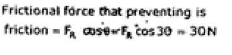## GATE Mechanical (ME) 2023 Mock Test Series

27 docs|243 tests
 Use Code STAYHOME200 and get INR 200 additional OFF Use Coupon Code